Examples

Chapter 6 Class 12 Application of Derivatives
Serial order wise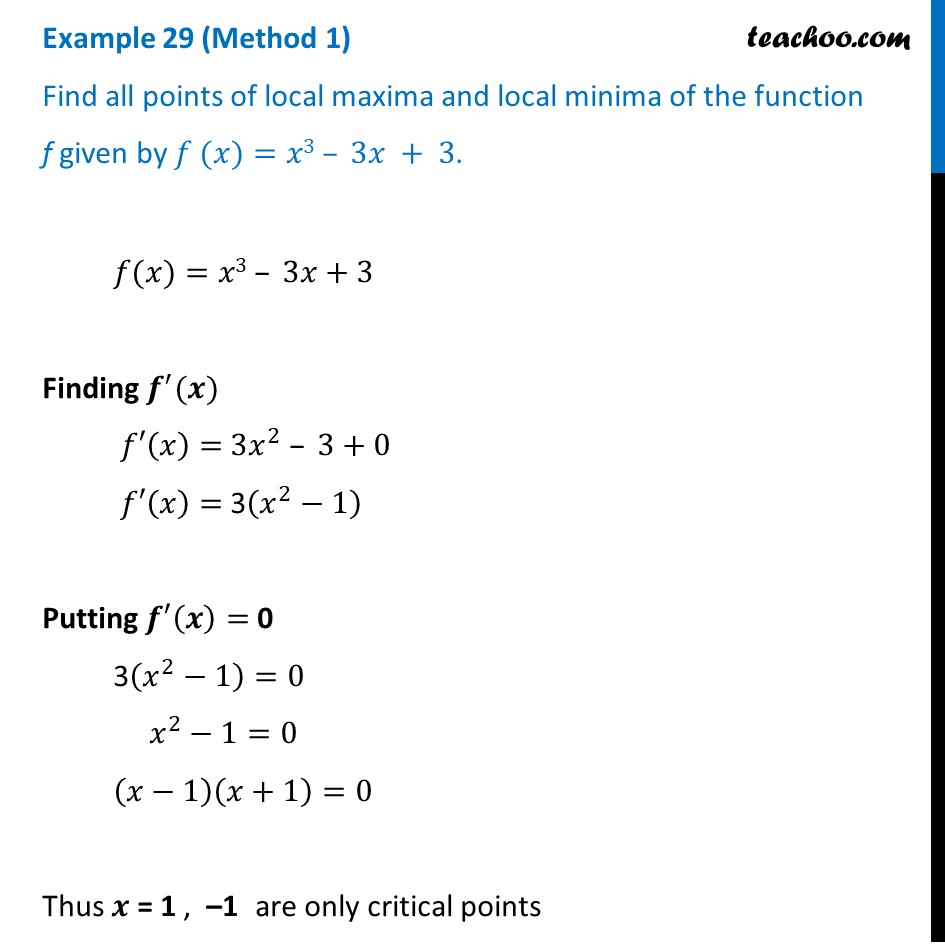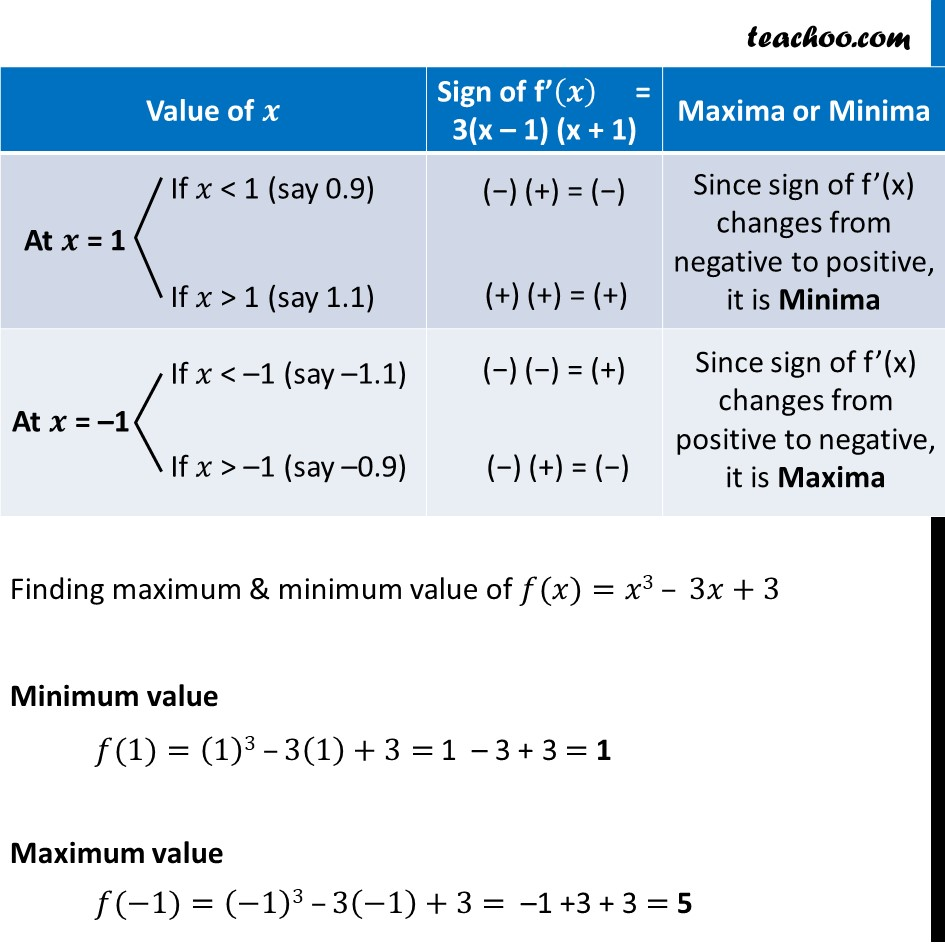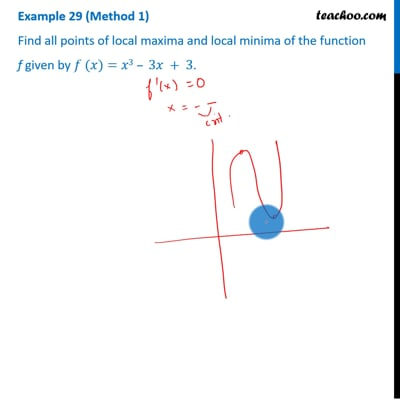This video is only available for Teachoo black users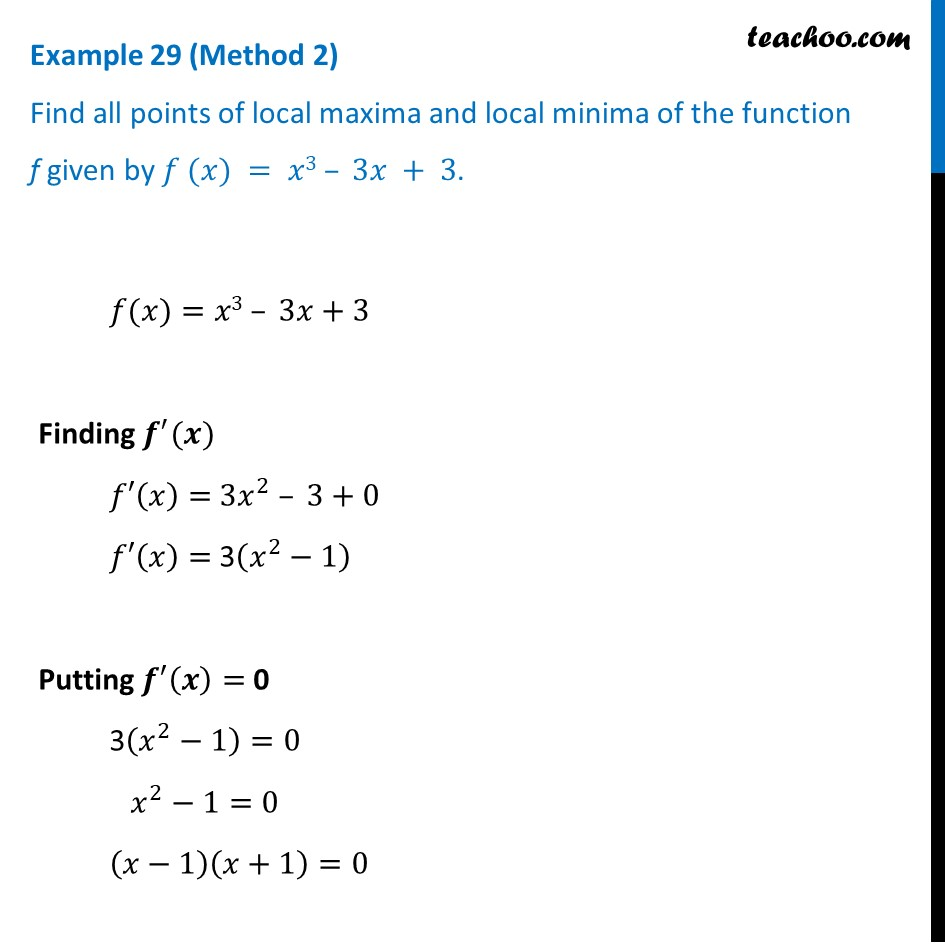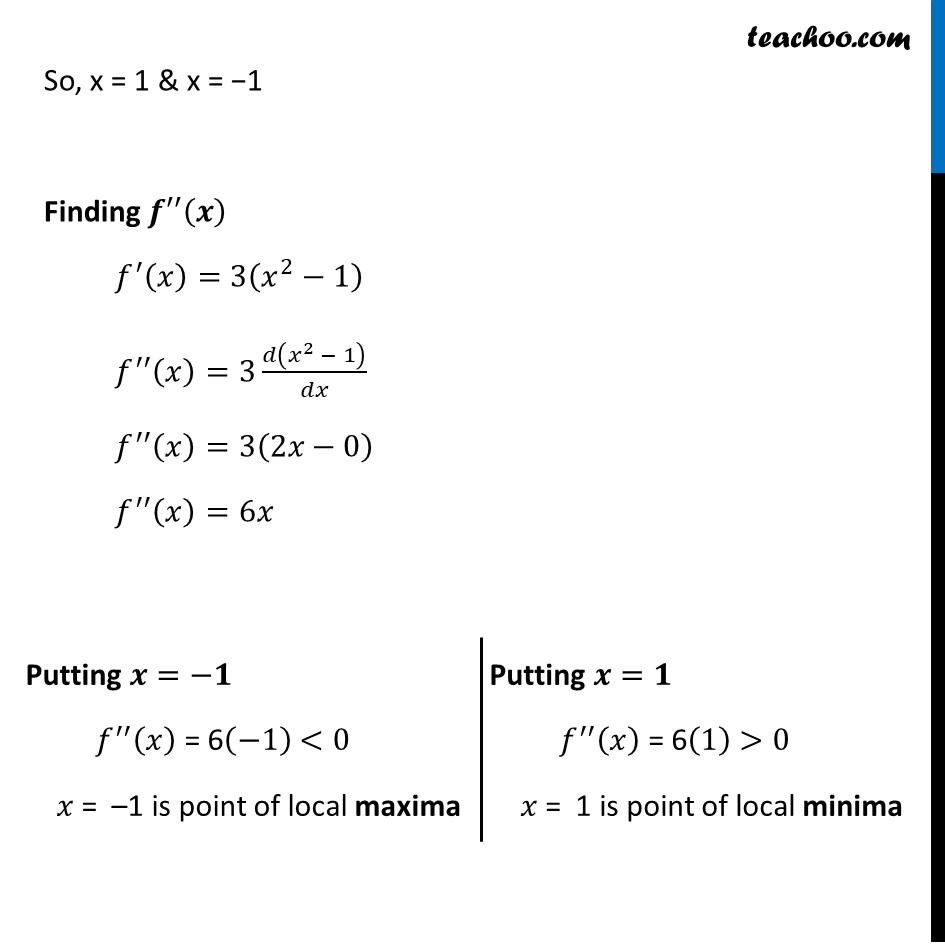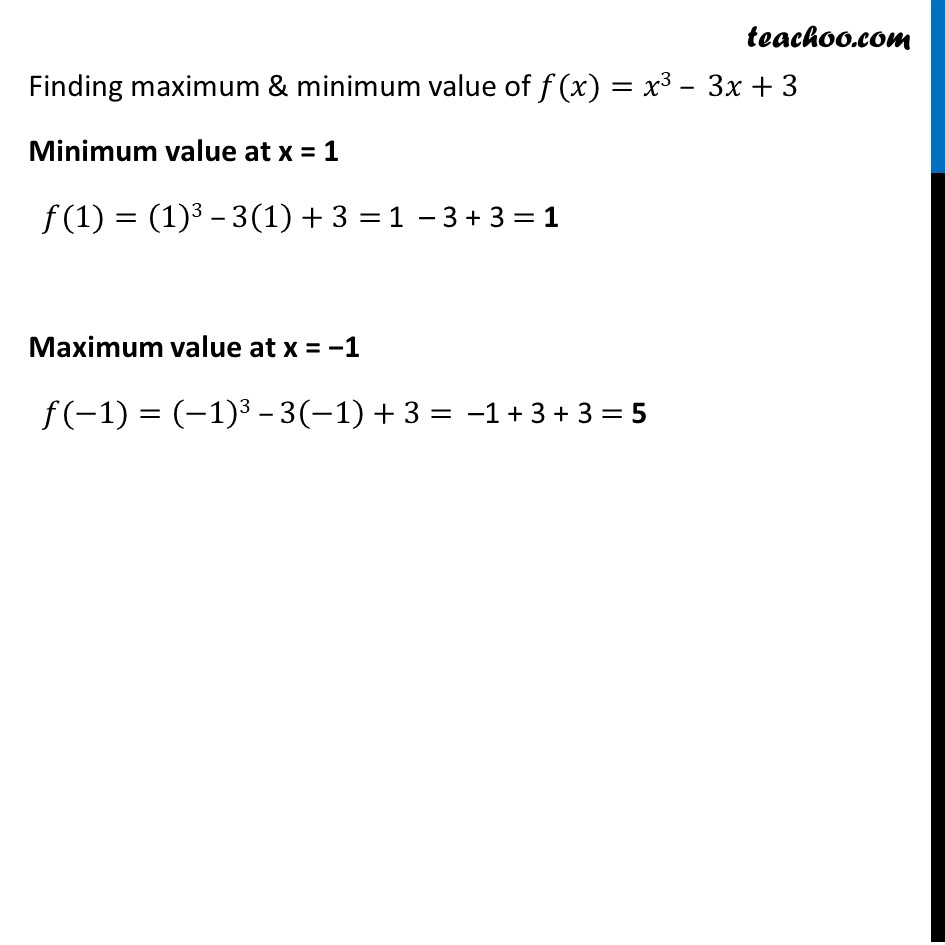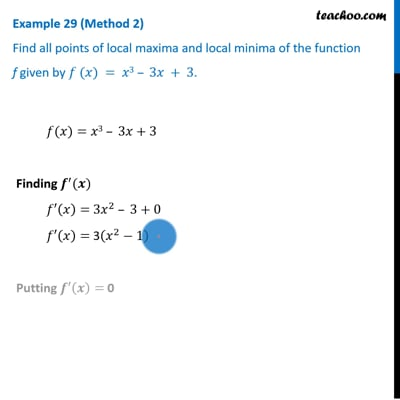This video is only available for Teachoo black users

Solve all your doubts with Teachoo Black (new monthly pack available now!)

### Transcript

Example 29 (Method 1) Find all points of local maxima and local minima of the function f given by 𝑓 (𝑥)=𝑥3 – 3𝑥 + 3. 𝑓(𝑥)=𝑥3 – 3𝑥+3 Finding 𝒇^′ (𝒙) 𝑓′(𝑥)= 3𝑥^2 – 3+0 𝑓′(𝑥)= 3(𝑥^2−1) Putting 𝒇′(𝒙)= 0 3(𝑥^2−1)=0 𝑥^2−1=0 (𝑥−1)(𝑥+1)=0 Thus 𝒙 = 1 , –1 are only critical points Finding maximum & minimum value of 𝑓(𝑥)=𝑥3 – 3𝑥+3 Minimum value 𝑓(1)=(1)3 –3(1)+3= 1 – 3 + 3 = 1 Maximum value 𝑓(−1)=(−1)3 –3(−1)+3= –1 +3 + 3 = 5 Example 29 (Method 2) Find all points of local maxima and local minima of the function f given by 𝑓 (𝑥) = 𝑥3 – 3𝑥 + 3. 𝑓(𝑥)=𝑥3 – 3𝑥+3 Finding 𝒇^′ (𝒙) 𝑓′(𝑥)= 3𝑥^2 – 3+0 𝑓′(𝑥)= 3(𝑥^2−1) Putting 𝒇′(𝒙)= 0 3(𝑥^2−1)=0 𝑥^2−1=0 (𝑥−1)(𝑥+1)=0 So, x = 1 & x = −1 Finding 𝒇′′(𝒙) 𝑓^′ (𝑥)=3(𝑥^2−1) 𝑓^′′ (𝑥)=3 𝑑(𝑥^2 − 1)/𝑑𝑥 𝑓^′′ (𝑥)=3(2𝑥−0) 𝑓^′′ (𝑥)=6𝑥 Putting 𝒙=−𝟏 𝑓^′′ (𝑥) = 6(−1) <0 𝑥 = –1 is point of local maxima Putting 𝒙=𝟏 𝑓^′′ (𝑥) = 6(1) >0 𝑥 = 1 is point of local minima Finding maximum & minimum value of 𝑓(𝑥)=𝑥3 – 3𝑥+3 Minimum value at x = 1 𝑓(1)=(1)3 –3(1)+3= 1 – 3 + 3 = 1 Maximum value at x = −1 𝑓(−1)=(−1)3 –3(−1)+3= –1 + 3 + 3 = 5